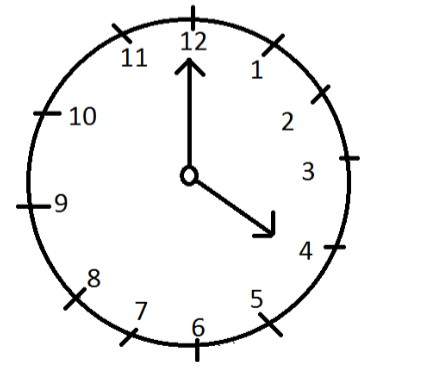QuestionAnswers

# At what time between, four o’clock & five o’clock, are the hands $2$minutes space apart?Verified
129.9k+ views
Hint: Clock concept: - The dial of the clock is circular in shape and was divided into $60$equal minute space.
$60$minute space traces an angle ${360^0}$
$1$minute space traces an angle ${60^0}$.

Complete step by step solution:
Minute spaces: The face or dial of watch is a circle whose circumference is divided into $60$equal parts, called minute spaces.
Hour hand & Minute hand: A clock has two hands, the smaller one is called the hour hand or shorthand, while the larger one is called minute hand or long hand.
In $60$equal minutes, the minute hand gains $55$minutes on the hour on the hour hand.
When the two hands are at right angles, they are $15$minutes spaces apart.
Angle traced by hour hand in $12$hours $= {360^0}$.
Angle traced by minute hand in $60$min $= {360^0}$.
Therefore,At 4 o’clock the two hands are 20-minute spaces apart.

To be in 2 minute, they must be 2 minute spaces apart.
Minute hand will have to gain $\left( {20 + 2 = 22} \right)$minute spaces.
As we know that,
$55$minute spaces are gained in $60$minute.
$22$minute spaces are gained in $\dfrac{{60}}{{55}} \times 2$minute$= 24$minute.
Hence the hands will be $2$minute apart at $24$minute past.

Note: If the clock or watch indicates $6$hour $10$minute when the correct time is $6$, it is said that the clock is $10$minute too fast.
If it indicates $6:40$when the correct time is $7$, it is said to be $20$min slow.